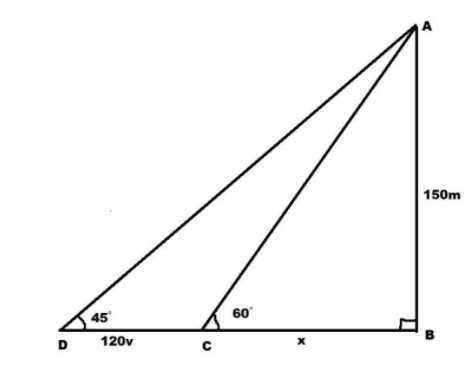QuestionAnswers

# A man in a boat rowed away from a cliff $150$ meters high takes $2$ minutes to change the angle from ${60^ \circ }$ to ${45^ \circ }$. The speed of the boat isVerified
130.8k+ views
Hint:Draw the diagram according to the given data. Use the relation between the speed, time and distance to complete the diagram.Later on, use simple trigonometric formulae to form equations and solve these equations to get the result.

Let us note down the values that we are given in the data,
Height of the cliff is $150m$.
Time taken to change angle is $2$ minutes. But it's better to use standard units seconds. So, we convert minutes into the seconds. We know that one minute will be equal to 60 seconds.
So, time taken to change the angle in seconds is $120$ seconds.
So, to get the distance we are going to use the relation between the speed, time and distance.
As distance is equal to the speed multiplied with the time, and assume the speed to be $v$
Here it will be $120v$ meters.
Angle variation is from ${60^ \circ }$ to ${45^ \circ }$. As angle is reduced,the man will move away from the cliff.
Now, let us draw the diagram according to the given data,Look at the $\Delta ABC$,
We need to find the value of unknown $x$,
To do so we need to use $\tan \theta$ and it is equal to as follows:
$\tan \theta = \dfrac{{{\text{Opposite}}}}{{Adjacent}}$
Hence $\tan {60^ \circ } = \dfrac{{150}}{x}$
We also know that the value of $\tan {60^ \circ }$ is $\sqrt 3$. Substitute in the above formula to get the value of $x$
$\Rightarrow \sqrt 3 = \dfrac{{150}}{x} \\ \Rightarrow x = \dfrac{{150}}{{\sqrt 3 }} \\ \Rightarrow x = 86.6m \\$
Now look at the $\Delta ABD$,
To find out the value of $v$ with known $x$ value, we need to use $\tan \theta$ once again
Hence $\tan {45^ \circ } = \dfrac{{150}}{{120v + x}}$
Substitute $\tan {45^ \circ } = 1$ and $x = 86.6$ to get the value of $v$.
$\Rightarrow 1 = \dfrac{{150}}{{120v + 86.6}} \\ \Rightarrow 120v + 86.6 = 150 \\ \Rightarrow 120v = 150 - 86.6 \\ \Rightarrow 120v = 63.4 \\ \Rightarrow v = \dfrac{{63.4}}{{120}} \\ \Rightarrow v = 0.52m/s \\$
So, the speed of the boat is $0.52m/s$.

Note:When you see this kind of problem, it’s better to draw the diagram first.It can be solved easily by using some basic trigonometric formulae.There would be some way to find out the length as we did using relation between speed, time and distance in this problem.In this way correlate all the unknown terms to form the equations with unknown values of variables. After solving these equations we will get the solution.Students should know the formula of trigonometric ratios and standard trigonometric angles i.e $\tan {45^ \circ } = 1$ and $\tan {60^ \circ }$ is $\sqrt 3$ for solving these types of questions.